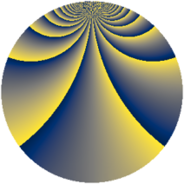# Properties

 Label 966.2.tLevel $966$ Weight $2$ Character orbit 966.t Rep. character $\chi_{966}(41,\cdot)$ Character field $\Q(\zeta_{22})$ Dimension $640$ Newform subspaces $1$ Sturm bound $384$ Trace bound $0$

# Related objects

## Defining parameters

 Level: $$N$$ $$=$$ $$966 = 2 \cdot 3 \cdot 7 \cdot 23$$ Weight: $$k$$ $$=$$ $$2$$ Character orbit: $$[\chi]$$ $$=$$ 966.t (of order $$22$$ and degree $$10$$) Character conductor: $$\operatorname{cond}(\chi)$$ $$=$$ $$483$$ Character field: $$\Q(\zeta_{22})$$ Newform subspaces: $$1$$ Sturm bound: $$384$$ Trace bound: $$0$$

## Dimensions

The following table gives the dimensions of various subspaces of $$M_{2}(966, [\chi])$$.

Total New Old
Modular forms 2000 640 1360
Cusp forms 1840 640 1200
Eisenstein series 160 0 160

## Trace form

 $$640q + 64q^{4} + 8q^{9} + O(q^{10})$$ $$640q + 64q^{4} + 8q^{9} + 8q^{15} - 64q^{16} + 20q^{18} + 54q^{21} - 8q^{22} - 56q^{25} - 44q^{30} - 8q^{36} + 20q^{37} - 16q^{39} - 4q^{42} - 28q^{43} + 24q^{46} - 68q^{49} - 40q^{51} + 32q^{57} - 80q^{58} - 8q^{60} + 86q^{63} + 64q^{64} + 24q^{67} + 72q^{70} - 64q^{72} - 8q^{78} - 144q^{79} + 20q^{81} + 34q^{84} + 32q^{85} + 8q^{88} - 40q^{91} + 16q^{93} + 68q^{99} + O(q^{100})$$

## Decomposition of $$S_{2}^{\mathrm{new}}(966, [\chi])$$ into newform subspaces

Label Dim. $$A$$ Field CM Traces $q$-expansion
$$a_2$$ $$a_3$$ $$a_5$$ $$a_7$$
966.2.t.a $$640$$ $$7.714$$ None $$0$$ $$0$$ $$0$$ $$0$$

## Decomposition of $$S_{2}^{\mathrm{old}}(966, [\chi])$$ into lower level spaces

$$S_{2}^{\mathrm{old}}(966, [\chi]) \cong$$ $$S_{2}^{\mathrm{new}}(483, [\chi])$$$$^{\oplus 2}$$